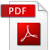### COURSE OUTCOME

COURSE OUTCOME

Sr. No.

Department

Description

1 BOTANY Statements of program Specific Outcomes (PSOs) & course outcomes (Cos) of B.Sc BOTANYStatements of program me Specific Outcomes (PSOs) & course outcomes (Cos) of M.Sc BOTANY2 CHEMISTRY Statements of program Specific Outcomes (PSOs) & course outcomes (Cos) of B.Sc CHEMISTRYStatements of program me Specific Outcomes (PSOs) & course outcomes (Cos) of M.Sc CHEMISTRY3 ENVIRONMENTAL SCIENCE Statements of program Specific Outcomes (PSOs) & course outcomes (Cos) of B.Sc ENVIRONMENTAL SCIENCEStatements of program me Specific Outcomes (PSOs) & course outcomes (Cos) of M.Sc ENVIRONMENTAL SCIENCE4 PHYSICS Statements of program Specific Outcomes (PSOs) & course outcomes (Cos) of B.Sc PHYSICSStatements of program me Specific Outcomes (PSOs) & course outcomes (Cos) of M.Sc PHYSICS5 MATHEMATICS Statements of program Specific Outcomes (PSOs) & course outcomes (Cos) of B.Sc MATHEMATICSStatements of program me Specific Outcomes (PSOs) & course outcomes (Cos) of M.Sc MATHEMATICS6 STATISTICS Statements of program Specific Outcomes (PSOs) & course outcomes (Cos) of B.Sc STATISTICSStatements of program me Specific Outcomes (PSOs) & course outcomes (Cos) of M.Sc STATISTICS7 ZOOLOGY Statements of program Specific Outcomes (PSOs) & course outcomes (Cos) of B.Sc ZOOLOGYStatements of program me Specific Outcomes (PSOs) & course outcomes (Cos) of M.Sc ZOOLOGY8 COMPUTER SCIENCE Statements of program Specific Outcomes (PSOs) & course outcomes (Cos) of B.Sc COMPUTER SCIENCE9 ELECTRONICS Statements of program me Specific Outcomes (PSOs) & course outcomes (Cos) of M.Sc ELECTRONICS